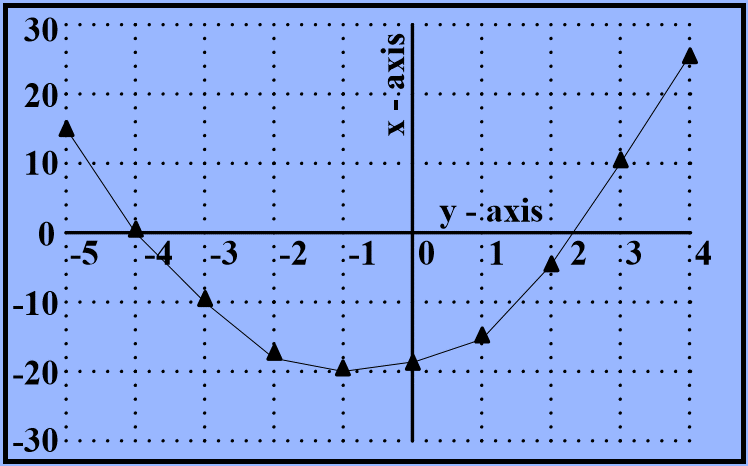# XAT 2015 Quant & DI: Quadratic Equations

This question appeared as a part of the quantitative reasoning and data interpretation section of the XAT 2015. Question 8 of a total of 33 questions that appeared in this section in XAT 2015. This one is an easy aptitude / quant question that tests concepts about quadratic equations and the curves that these equations represent.

## Question

Find the equation of the graph shown below.1. y = 3x - 4
2. y = 2x2 - 40
3. x = 2y2 - 40
4. y = 2x2 + 3x - 19
5. x = 2y2 + 3y - 19

Correct Answer      Choice E. x = 2y2 + 3y - 19

• #### Given Data

In the graph, x and y-axes have been swapped from the conventional ones.
i.e,. horizontal axis has been named y-axis and the vertical axis has been named x-axis.

#### Key Inference

The curve is a parabola.
A parabola will have an equation of the form y = ax2 + bx + c
However, in our case, the x and y axes have been swapped.
So, the equation has to be of the form x = ay2 + by + c

#### Points of Interest

To determine which of the equations fits the curve given, we are going to substitute values of x or y from the graph in the equations and check for a match. We need two to three points to determine that. Let us select points where at least one of the coordinates is 0. The points of interest therefore are:
1. When y is around -4, x = 0
2. When y is between 2 and 3, x = 0
3. When y is 0, x is around -20

#### What is the approach?

Substitute x = 0 in the choices that match the form of equation stated in the key inference step and see which one matches the data.
Cross check with another value when y = 0.
The choice that matches both conditions is the answer.

• #### Compute the equation

The equation has to be of the form x = ay2 + by + c.
A quick look at the choices tells us that we can eliminate choices A, B, and D.
Choice A is a linear equation. So, it represents a straight line. Rule it out.
Choices B and D are of the form y = ax2. Our axes are swapped. So, choice B can also be ruled out.

Choice C: x = 2y2 – 40
Substitute y = -4 in the equation.
We get x = 2(-4)2 – 40 = 32 – 40 = -8.
However, we know that when y = -4, x = 0.

So, Choice C cannot be the answer.

Choice E: x = 2y2 + 3y – 19
When y = -4, x = 2(-4)2 +3(-4) – 19 = 32 – 31 = 1.
So, when y is around -4, it will be 0.

Let us validate it with one more data point.
When y = 0, x = 2(0)2 + 3(0) – 19 = -19.
The value of x is around -20 as indicated in the curve.

The correct answer is Choice E.

## Online TANCET MBA CourseTry it Free!

Register in 2 easy steps and start learning in 5 minutes!